### What Are Summary Tasks ?

Instead of having to look at all the tasks individually, summary tasks allow users to view information about groups of tasks all at once. Summary tasks have subtasks , and the summary task is, as the name implies, a summary of the subtasks under it. The start date of a summary task is equal to the earliest start date of any of its subtasks. Likewise, its finish date is the latest of all the finish dates of its subtasks. Other fields, such as Work and Cost, are the sum of all the subtasks' Work and Cost fields. Because the summary task summarizes the information about its subtasks, it can be very useful when examining how much is to be done on a specific part of the project, or how much has already been done.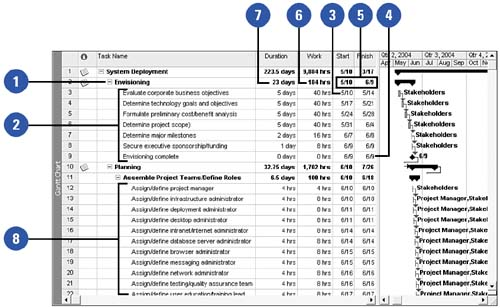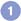The Envisioning task is a summary task.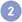The tasks with IDs 3 through 9 are subtasks of the Envisioning task.The earliest Start date among the subtasks of Envisioning is 5/10.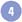The latest Finish date among the subtasks is 6/9.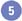These dates roll up to the Start and Finish dates for Envisioning.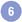The sum of all the Work fields for the subtasks of Envisioning is 184 hours.The Duration field for a summary task is calculated just like the duration of a normal task ”it is the number of working days between the start and finish dates for the task. It can often appear, as in this example, that it is the sum of the subtasks' Duration fields, but this is not the case.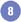Summary tasks can be nested ; that is, one summary task can have other summary tasks as subtasks. For example, the task Assemble Project Teams/Define Roles is a subtask of the Planning Summary task, but is itself the summary task of Task IDs 12 “24.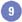Summary tasks can be collapsed to hide their subtasks. The plus sign to the left of a summary task's name shows that its subtasks are hidden.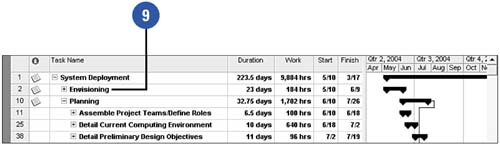Show Me Microsoft Office Project 2003
ISBN: 0789730693
EAN: 2147483647
Year: 2003
Pages: 204